## Monday, 13 August 2012

### An open problem, matlab and randomness II

Before we deal with the original problem again, let's deal with the following problem:

Given X as a uniform distribution from 0 to 1. What's the expected value of E(1/X)?

Recall the formula of E(X) for uniform distribution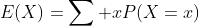When we extend the formula into continous distribution it becomes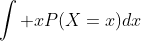.

Firstly we have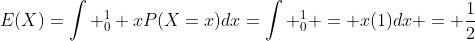.

Now how can we get the probability distribution for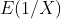?

Consider the following, let a and b be numbers within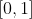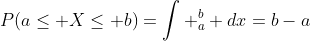Now take the inverse, let the inverse of X be Y: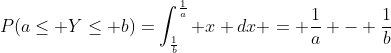. It's hard to deal with the integral form, but consider the probability density function with limits. Applying idea from calculus,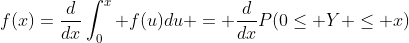which is FToC II (Fundamental Theorem of Calculus).

## Friday, 3 August 2012

### An open problem, matlab and randomness

Just sharing a question that I've done recently, and that actually recalls something that I intend to write some times before but gave up very soon.

Now consider a hypothetical cat and a mouse on a circle chasing each other.
1) Cat's speed is random from -2 to 2 (-ve = anti-clockwise)
2) Mouse's speed is i) fixed at 1 ii) random within a certain range.
3) The cat "catches" the mouse if the cat "catches" the mouse or the mouse "catches" the cat.
4) The mouse starts at random position of circle (radius 2) while cat starts at x=0.

And let's deal with the matlab code...
-------------
clear
vm=zeros(401,1000)+1; %vm = speed of mouse, we are generating a matrix here in case we will use random speed later
d=rand(401,1000)*4*pi %random position of mouse
A=zeros(401,1000);
for I=1:401
vc=(I-201)/100;
for J=1:1000
if vc > vm(I,J)
A(I,J)=abs(vc*d(I,J)/(vc-vm(I,J));
else
A(I,J)=abs(vc*(4*pi-d(I,J))/(vc-vm(I,J));
end
end
end
for I=1:201
y(I)=(mean(A(200+I,:))+mean(A(202-I,:)))/2;
end
%direction of cat is the not our concern (though it behaves differently)
y(101)=(y(100)+y(102))/2;
%If vc=vm the cat never catches the mouse and by default distance travelled = 0 is not our interest, so delete that entry and smooth the curve.
x=0:0.01:2;
plot(x,y)
----------
Output:

(In fact I'm not using y(101) as the mean of y(100) and y(102) here because it's supposed to tend to infinity and hence it's not the mean of the neighbouring terms.)

What can you observe? A significant peak like the IR spectrometry or something else? Even though we are generating randomness, this is pretty much an "expected" result --- let see what we can interpret here.